System trading starting with Python3: long-term investment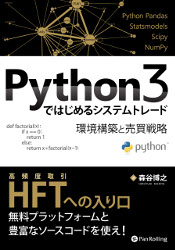Basics of long-term investment

In this article, you will learn the most basic data analysis methods with long-term investment in mind.

--Analyzing the characteristics of price movements with data available over the long term --Understanding the market size --Fake trends and random walks --To avoid bankruptcy

Analyzing the characteristics of price movements with long-term data

Do you think that it is better to use the latest data for proper analysis? I sometimes hear that the stock market is a living thing and its nature changes, so it should be analyzed with short data. But that's a mistake. With this kind of analysis method, the analysis result that you are expecting will come out immediately. The data used for the data has never been so long. It's never too long. Sometimes it is necessary to create pseudo data using the Monte Carlo method.

Understanding the market size

The basis of long-term investment is to know the other party well. It starts with comparing with others. Make sure you understand the exact size of the market when making an investment.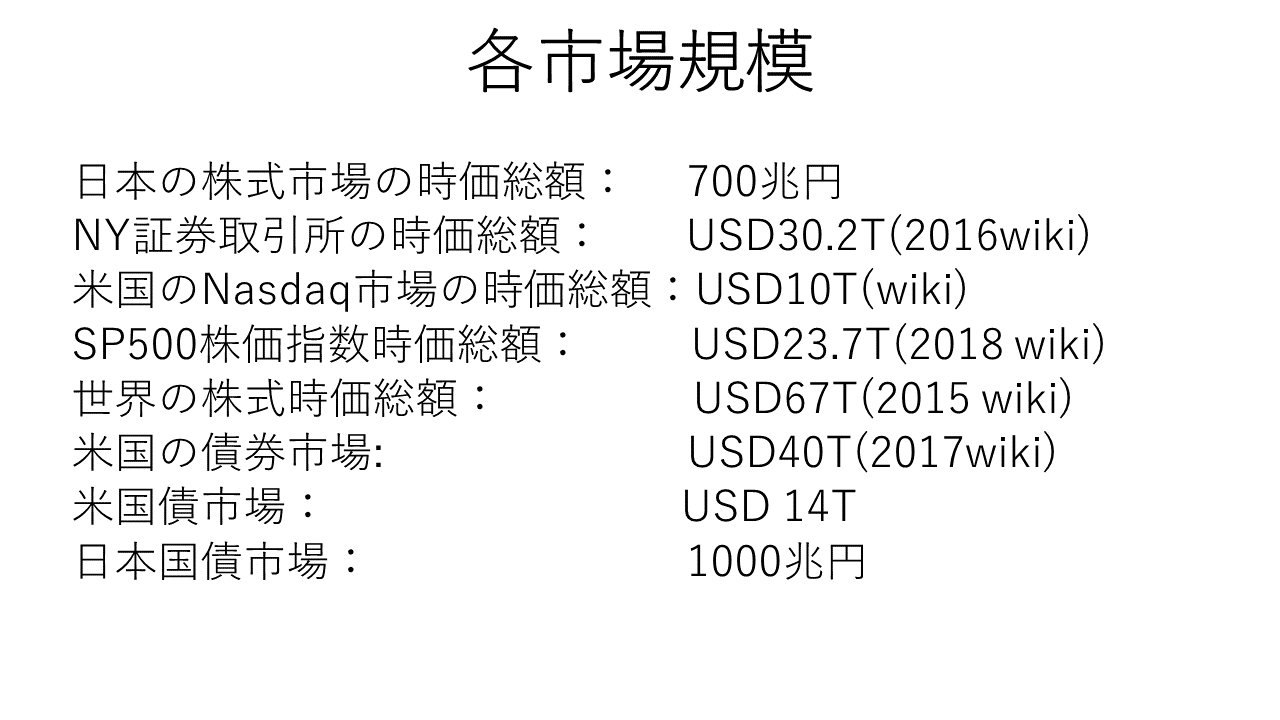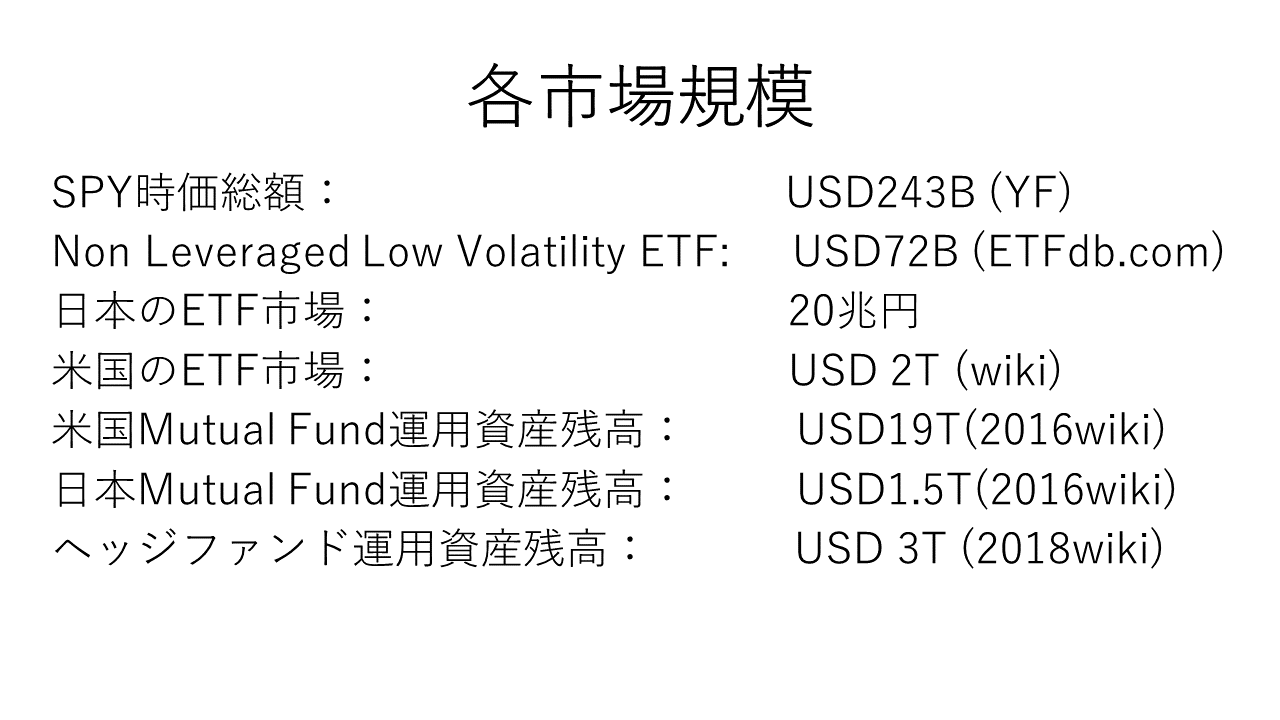Did you get a sense of scale? At least the US financial markets are bigger than Japan. And while the Japanese government bond market is larger than the stock market, it is not the case in the United States. Given that GDP per capita is the same in both countries, which stock market is more likely to rise? I think that various images can be created with just this analysis.

Fake trends and random walks

Many economic time series and stock prices follow a random walk. The mean and variance of the time series that follow the random walk will change over time. In a random walk, the mean changes stochastically over time, and the variance increases in proportion to the square root over time. Therefore, the future of the stock price is unpredictable. The fact that you cannot predict is meaningless even if you plan the timing of buying and selling. You don't know where to go in the future, as the random walk is likened to a drunken staggered foot. And the width increases with the passage of time. In other words, it may rise significantly, but it will also fall.

Knowing whether stock data follows a random walk is important in two ways:

1. Is the trend that occurred a fake trend, or is the trend happening?
2. When the two time series show a correlation, are they a fake correlation or do they happen to occur? That is the point.

Let's create a random walk in Python. Next, the figure shows the downward movement made by random numbers. It is falling in almost one direction.

%matplotlib inline
import matplotlib.pyplot as plt
import numpy as np
import pandas as pd
def generate(y0,rho,sigma,nsample):
e = np.random.normal(size=nsample)
y=[]
y.append(rho*y0+e)
for i,u in enumerate(e[1:]):
y.append(rho*y[i]+u)
plt.plot(y)
generate(1,1,1,100)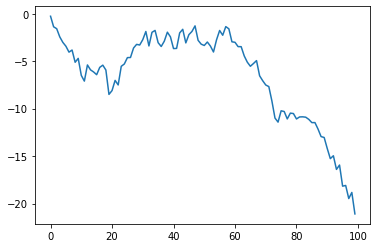Next, let's look at the actual stock price. The Dickey-Fuller test is a way to determine if a stock price is a random walk. For this test

--Random walk without drift (rw) --Random walk with drift (drw) --Random Shok with Time Trend Drift (tdrw) --Random walk with time trend drift with acceleration (qtdrw)

there is. If these results are less than or equal to 0.1, it is more likely that you are not a random walk.

Let's actually take a look at the minimum variance portfolio (usmv), NASDAQ 100 (qqq), S & P500 (spy), and Dow Jones Industrial Average (dia).

BASIC=['usmv','qqq','spy','dia']

#ror.plot()
ror = ror/250/100#jpy

def LongtermInvest(PORT,ror,start):
i=1
for asset in PORT:
dtsd=tsd.pct_change()
tsda=tmp.iloc[:,2].cumprod()
ts=np.log(tsda.dropna())
ts.plot(label=str(asset))
if i==5:
plt.legend()
plt.show()
i=0
i+=1
if i!=1:
plt.legend()
plt.show()
i=1
LongtermInvest(BASIC,ror,'2015/1/4')
usmv (0.9545542347136421, 23) (0.8638006294387173, 23) (0.0012513095084584341, 11) (0.005811135279764244, 11)
qqq (0.9917530792757916, 9) (0.9725398688611976, 9) (0.13498405344227182, 9) (0.09924300082908494, 9)
spy (0.8831775184401023, 9) (0.8368954755934965, 9) (0.00474390277885088, 9) (0.018407642576579095, 9)
dia (0.8438357944084672, 10) (0.7816330208711864, 10) (0.05496092342671481, 9) (0.08039004988307125, 9)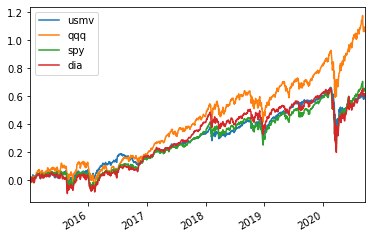The results are, in order, a random walk without drift, a random walk with drift, a random show with time trend drift, and a random walk with time trend drift with acceleration. The results suggest that there may be a time trend (tdrw, qtdrw) in all cases.

To avoid bankruptcy

Which of the four basic equity trading strategies has the lowest probability of bankruptcy?

--Rebalancing: A strategy to restore the investment ratio if it deviates from the target value due to price movements. --Loss cut: When a loss occurs, close the position. --Option: Pay a premium like insurance to avoid risk. --Buy and Hold: Continue to hold a buy position.

Of these, the only strategies with a low probability of bankruptcy are rebalancing and buying.

--Options will go bankrupt if profits commensurate with the option fee are not obtained. --The loss cut will go bankrupt if the loss is larger than the profit.

Let's simulate using Apple stock and Intel stock. The following program is a simulation of the case where you do not move your position after first investing in half. In this case, the ratio of the value of the investment will be higher for the stock with the higher stock price.

aapl=aapl/aapl.iloc#Indexing of stock prices
lnaapl=np.log(aapl)
dlnaapl=lnaapl.diff().dropna()
intc=intc/intc.iloc
intc
lnintc=np.log(intc)
dlnintc=lnintc.diff().dropna()
lnaapl.plot(label='apple',style='-.')
lnintc.plot(label='intel',linestyle='--')
lnport=0.5*lnaapl+0.5*lnintc
lnport.plot(label='no rebalance')
plt.legend(loc='upper left')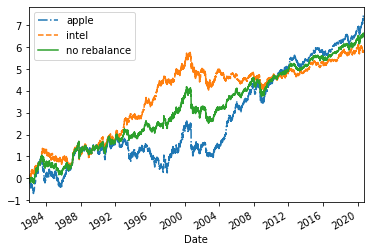The following simulation is a program that rebalances daily and always adjusts the value of the investment to a ratio of 50% 50%.

def portfolio_rebalance(tsd1,tsd2):
port=pd.concat([tsd1,tsd2],axis=1).dropna()
port.columns=('p1','p2')
port['a1']=0
port['a2']=0
port['v']=1
n=len(port)
p1=port['p1'].iloc
p2=port['p2'].iloc
v=port['v'].iloc
a1=float(v/2/p1)
a2=float(v/2/p2)
port.iloc[0,2]=a1
port.iloc[0,3]=a2
for i in range(1,len(port)):
p1=port['p1'].iloc[i]#Apple stock price today
p2=port['p2'].iloc[i]#Intel stock price today
p1_0=port['p1'].iloc[i-1]#Apple stock price the day before
p2_0=port['p2'].iloc[i-1]#Intel stock price the day before
a1_0=port['a1'].iloc[i-1]#Number of shares held by Apple the day before
a2_0=port['a2'].iloc[i-1]#Number of Intel holdings the day before
v_0=port['v'].iloc[i-1]#Value of the previous day's rebalancing portfolio
#v=a1_0*(p1-p1_0)+a2_0*(p2-p2_0)+v_0#Value of today's rebalancing portfolio
v=a1_0*p1+a2_0*p2#Value of today's rebalancing portfolio
port.iloc[i,4]=v#Rebalance Portfolio Value Update
port.iloc[i,2]=a1#Apple Stock Update
port.iloc[i,3]=a2#Intel share count update
port['v2']=0.5*port.p1+0.5*port.p2#Value of portfolio without rebalancing
return port
port=portfolio_rebalance(aapl,intc)
lnport=np.log(port)
lnport.v.plot(label="port daily rebalance",linewidth=1.0)
lnaapl.plot(label='apple',style='-.')
lnintc.plot(label='intel',linestyle='--')
plt.legend(loc="upper left")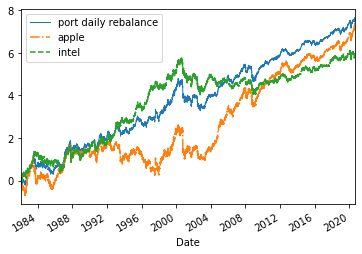I think you have an image of the investment efficiency of rebalancing.

Now, can this avoid bankruptcy? Even if you choose two stocks, the probability that two companies will go bankrupt is not zero. You can avoid that risk by investing in a stock index. This is why we encourage beginners to invest in stock indexes.

Is it possible to avoid bankruptcy with the dollar cost averaging method (funded investment)? Bankruptcy is avoided. Its investment efficiency is close to that of a "buy-and-hold" strategy. But what if the assets you invest in continue to fall for a long time? Don't you think it will put a lot of pressure on your heart? Consider the Nikkei average after the burst of the bubble, the gold market in the 1990s. Can you continue to invest in that asset if you lower it for more than 10 years in a row? If you can do that, the dollar cost averaging method is one of your strategies. However, I don't think the god of victory will smile at the investment method of this application, which is generally unwilling.